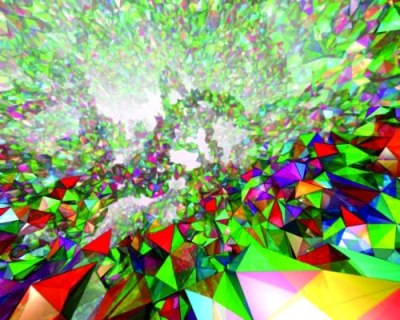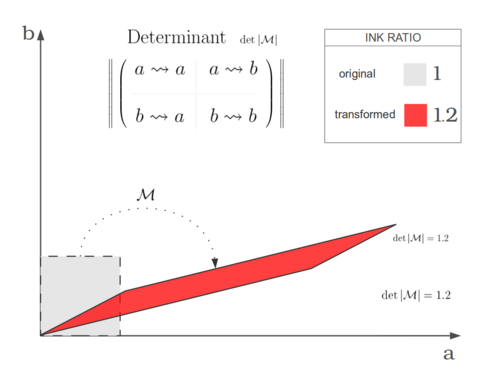### Linear Transformations will take you on a Trip Comparable to that of Magical Mushroom Sauce, And Perhaps cause More Lasting Damage

Long after I was supposed to “get it”, I finally came to understand matrices by looking at the above pictures. Staring and contemplating. I would come back to them week after week. This one is a stretch; this one is a shear; this one is a rotation. What’s the big F?

The thing is that mathematicians think about transforming an entire space at once. Any particular instance or experience must be of a point, but in order to conceive and prove statements about all varieties and possibilities, mathematicians think about “mappings of the entire possible space of objects”. (This is true in group theory as much as in linear algebra.)

So the change felt by individual ink-spots going from the original-F to the F-image would be the experience of an actual orbit in a dynamical system, of an actual feather blown by a bit of wind, an actual bullet passing through an actual heart, an actual droplet in the Mbezi River pulsing forward with the flow of time. But mathematicians consider the totality of possibilities all at once. That’s what “transforming the space” means.

What do the slots in the matrix mean? Combing from left to right across the rows of numbers often means “from”. Going from top to bottom along the columns often means “to”. This is true in Markov transition matrices for example, and those combing motions correspond with basic matrix multiplication.

So there’s a hint of causation to this matrix business. Rows are the “causes” and columns are the “effects”. Second row, fifth column is the causal contribution of input B to the resulting output E and so on. But that’s not 100% correct, it’s just a whiff of a hint of a suggestion of a truth.

The “domain and image” viewpoint in the pictures above (which come from Flanigan & Kazdan about halfway through) is a truer expression of the matrix concept.

• [ [1, 0], [0, 1] ] maps the Mona Lisa to itself,
• [ [.799, −.602], [.602, .799] ] has a determinant of 1 — does not change the amount of paint — and rotates the Mona Lisa by 37° counterclockwise,
• [ [1, 0], [0, 2] ] stretches the image northward;
• and so on.MATRICES IN WORDS

Matrices aren’t* just 2-D blocks of numbers — that’s a 2-array. Matrices are linear transformations. Because “matrix” comes with rules about how the numbers combine (inner product, outer product), a matrix is a verb whereas a 2-array, which can hold any kind of data with any or no rules attached to it, is a noun.

* (NB: Computer languages like `R`, Java, and `SAGE`/Python have their own definitions. They usually treat vector `==` list `&&` matrix `==` 2-array.)

Linear transformations in 1-D are incredibly restricted. They’re just proportional relationships, like “Buy 1 more carton of eggs and it will cost an extra \$2.17. Buy 2 more cartons of eggs and it will cost an extra \$4.34. Buy 3 more cartons of eggs and it will cost an extra \$6.51….”  Bo-ring.

In scary mathematical runes one writes:

And the property of linearity itself is written:

` `

The matrix revolution does so much generalisation of this simple concept it’s hard to imagine you’re still talking about the same thing. First of all, the insight that mathematically abstract vectors, including vectors of generalised numbers, can represent just about anything. Anything that can be “added” together.And I put the word “added” in quotes because, as long as you define an operation that obeys commutativity, associativity, and distributes over multiplication-by-a-scalar, you get to call it “addition”! See the mathematical definition of ring.

• The blues scale has a different notion of “addition” than the diatonic scale.
• Something different happens when you add a spiteful remark to a pleased emotional state than when you add it to an angry emotional state.
• Modular and noncommutative things can be “added”. Clock time, food recipes, chemicals in a reaction, and all kinds of freaky mathematical fauna fall under these categories.
• Polynomials, knots, braids, semigroup elements, lattices, dynamical systems, networks, can be “added”. Or was that “multiplied”? Like, whatever.
• Quantum states (in physics) can be “added”.But that’s just vectors. Matrices also add dimensionality. Linear transformations can be from and to any number of dimensions:

• 1→7
• 4→3
• 1671 → 5
• 18 → 188
• and X→1 is a special case, the functional. Functionals comprise performance metrics, size measurements, your final grade in a class, statistical moments (kurtosis, skew, variance, mean) and other statistical metrics (Value-at-Risk, median), divergence (not gradient nor curl), risk metrics, the temperature at any point in the room, EBITDA, not `function(x) { c( count(x), mean(x), median(x) ) }`, and … I’ll do another article on functionals.

In contemplating these maps from dimensionality to dimensionality, it’s a blessing that the underlying equation is so simple as linear (proportional). When thinking about information leakage, multi-parameter cause & effect, sources & sinks in a many-equation dynamical system, images and preimages and dual spaces; when the objects being linearly transformed are systems of partial differential equations, — being able to reduce the issue to mere multi-proportionalities is what makes the problems tractable at all.

So that’s why so much painstaking care is taken in abstract linear algebra to be absolutely precise — so that the applications which rely on compositions or repetitions or atlases or inversions of linear mappings will definitely go through.` `

Why would anyone care to learn matrices?

Understanding of matrices is the key difference between those who “get” higher maths and those who don’t. I’ve seen many grad students and professors reading up on linear algebra because they need it to understand some deep papers in their field.

• Linear transformations can be stitched together to create manifolds.
• If you add Fourier | harmonic | spectral techniques + linear algebra, you get really trippy — yet informative — views on things. Like spectral mesh compressions of ponies.
• The “linear basis” and “linear combination” metaphors extend far. For example, to eigenfaces or When Doves Cry Inside a Convex Hull.
• You can’t understand slack vectors or optimisation without matrices.
• JPEG, discrete wavelet transform, and video compression rely on linear algebra.
• A 2-matrix characterises graphs or flows on graphs. So that’s Facebook friends, water networks, internet traffic, ecosystems, Ising magnetism, Wassily Leontief’s vision of the economy, herd behaviour, network-effects in sales (“going viral”), and much, much more that you can understand — after you get over the matrix bar.
• The expectation operator of statistics (“average”) is linear.
• Dropping a variable from your statistical analysis is linear. Mathematicians call it “projection onto a lower-dimensional space” (second-to-last example at top).
• Taking-the-derivative is linear. (The differential, a linear approximation of a could-be-nonlinear function, is the noun that results from doing the take-the-derivative verb.)
• The composition of two linear functions is linear. The sum of two linear functions is linear. From these it follows that long differential equations—consisting of chains of “zoom-in-to-infinity” (via “take-the-derivative”) and “do-a-proportional-transformation-there” then “zoom-back-out” … long, long chains of this, can amount in total to no more than a linear transformation.• If you line up several linear transformations with the proper homes and targets, you can make hard problems easy and impossible problems tractable. The more “advanced-mathematics” the space you’re considering, the more things become linear transformations.
• That’s why linear operators are used in both quantum mechanical theory and practical things like building helicopters.
• You can understand dynamical systems, attractors, and thereby understand love better through matrices.